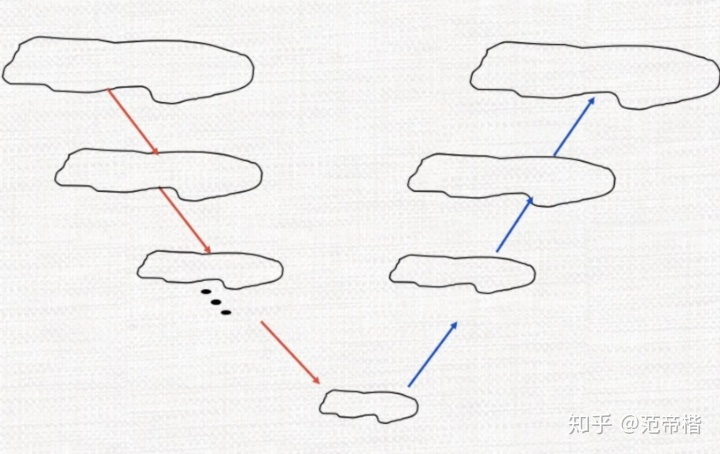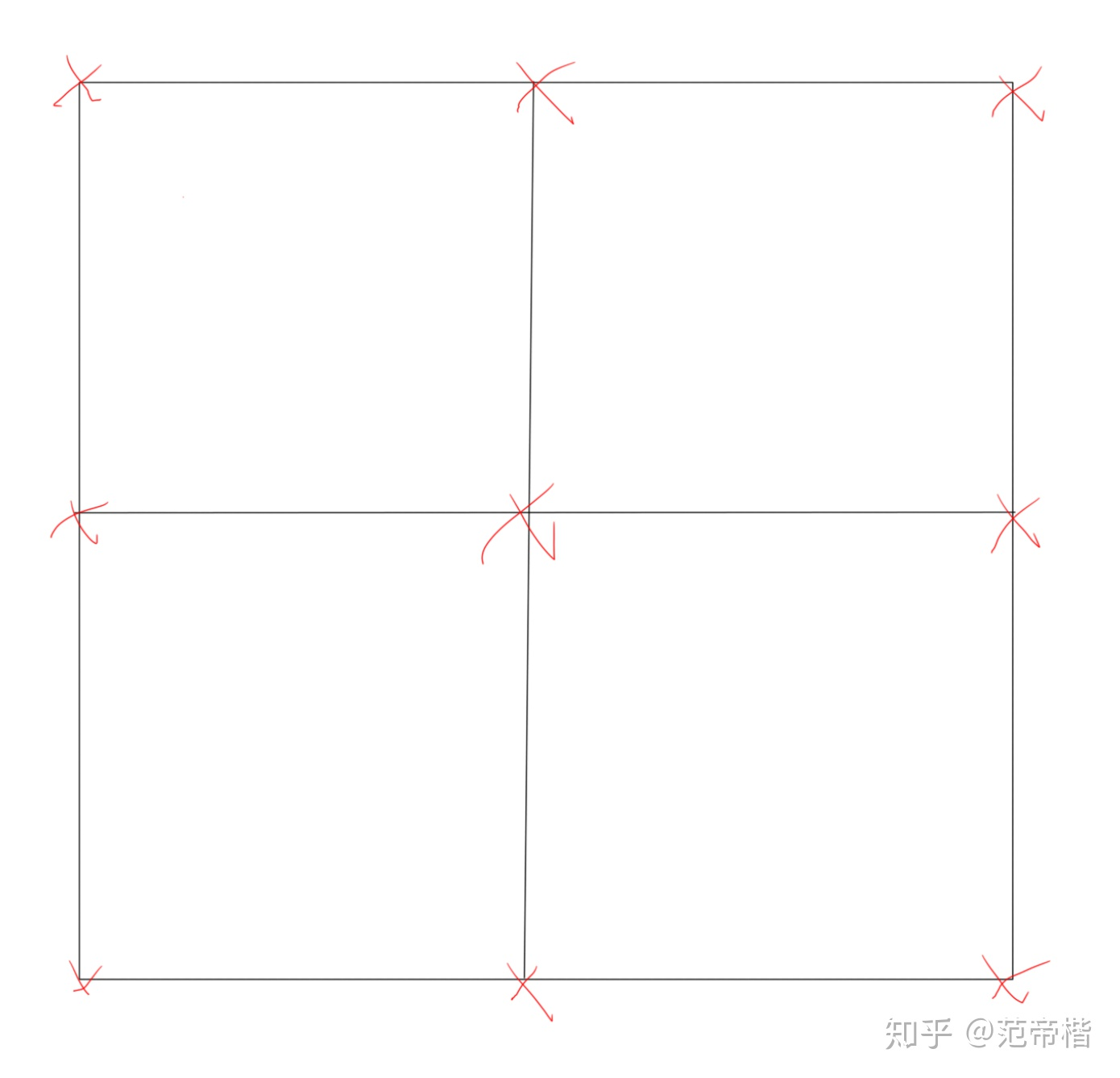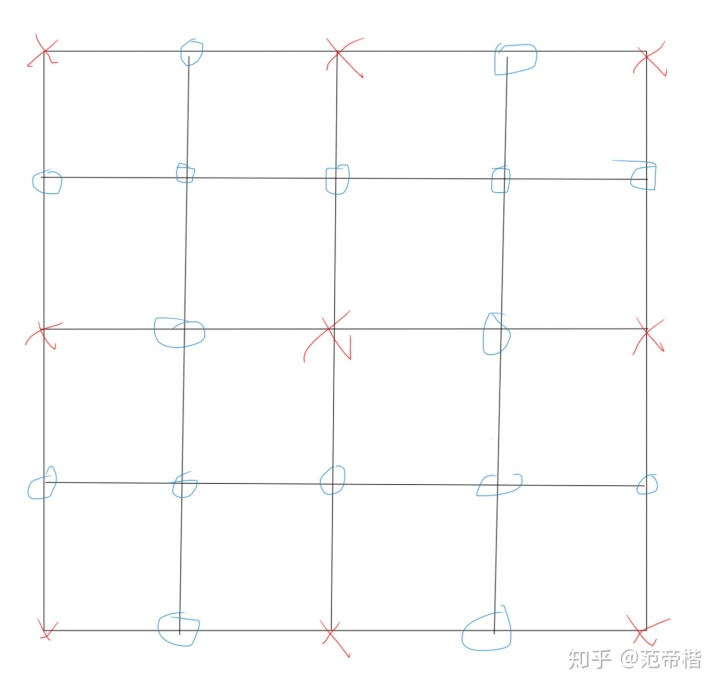• function [X_reality,n_reality] = ...% A为迭代的系数矩阵 % b为方程组右边的常数项（列向量） % X_start为迭代的初始向量 % n_limit为最大允许迭代的次数 % tolerance为精度上限值 %% % X_reality为最后结果...

function [X_reality,n_reality] = Jacobi(A,b,X_start,n_limit,tolerance)
%%
% A为迭代的系数矩阵
% b为方程组右边的常数项（列向量）
% X_start为迭代的初始向量
% n_limit为最大允许迭代的次数
% tolerance为精度上限值
%%
% X_reality为最后结果
% n_reality为最后迭代次数
%%
disp('雅克比迭代法求解线性方程组');
[n,n] = size(A);                 % A的行数和列数均为n
D = diag(diag(A));            % D的对角线元素根A的对角线元素相同，其余为0
B = inv(D) * (D - A);          % B为雅克比迭代矩阵，也就是化简后的便于迭代的等价方程组的系数矩阵
f  = inv(D) * b;                  % f为化简后的便于迭代的等价方程组的常数项向量
n_reality = 0;
%%
while 1
if(n_reality > n_limit)
disp('迭代次数超界');
break;
end
X_reality = B * X_start + f;                    % 雅可比迭代公式
n_reality = n_reality + 1;
if(norm(X_reality - X_start) <= tolerance)  % 如果满足条件||X(k+1) - X(k)||的2范数小于等于tolerance
break;                                             % 则退出函数
else
X_start = X_reality;                            % 循环迭代
end
end
end
%%

转载于:https://blog.51cto.com/592669550/932008
展开全文• 问题定义很多问题都可以抽象为求解下列优化的问题： 往往对于实际应用时，可能还会加入一些正则项，最终表示为求解优化： 这个问题有一个解析解： 因此上述问题等于求解线性方程组: 对于图像问题，一方面由于绝大...
问题定义很多问题都可以抽象为求解下列优化的问题：往往对于实际应用时，可能还会加入一些正则项，最终表示为求解优化：这个问题有一个解析解：因此上述问题等于求解线性方程组:对于图像问题，一方面由于绝大多数模型都只会建立某个像素与它局部之间的关系，因此线性方程组的系数矩阵通常满足某种特定的稀疏性；另一方面，由于图像分辨率较高，因此线性方程组维度实际上极大，导致快速计算难度较大。 因此，这个问题的最终核心在于：如何快速求解一个稀疏大规模的对称正定的线性方程组迭代法的阵亡对于简单迭代法，如雅可比迭代，高斯-塞德尔迭代等，都存在高频快速收敛，低频难以收敛的问题。 简单来说，对于柏松问题：这个问题在某种程度上等价于求解柏松方程离散化之后的线性方程组对应每一行的方程为(一维情况):对于上述问题使用雅可比松弛迭代(假设松弛变量为)，得到迭代矩阵的特征值和特征向量分别为(为向量维度,):其中表示第i个网格点的坐标 由此，可以发现，对于这个问题，设置为1，那么频率越高,k越大，越接近于0，收敛越快。 因此对于这类问题，往往高频的收敛性更好，低频收敛性更差。几何多重网格为了解决低频收敛慢的问题，多重网格算法应运而生。 核心思想在于，在细网格上的低频可以变为粗网格上的高频。 注意到对于，如果N变为N/2实际上可以认为频率变为了原来的两倍，那么收敛性自然会变好。 因此，我们可以先在细网格上迭代，消除了高频误差，然后放到粗网格迭代，消除细网格上的低频误差，然后再在粗网格上refine最终结果，大致流程如下图：这个方法实际上是比较简单的，网格如下所示：红色的点代表粗网格上的节点，如果我们将网格细化，那么，可以得到细网格的点：这些点的值通过粗网格插值而来，也就是说，几何多重网格实际上是依赖于实际的网格，人为设定了粗网格和细网格，从而设置的一种多重网格算法。注意到这种方法细网格上的节点上一定有粗网格上的节点，因此原来如果粗细网格原来是同一个点，只需要将粗网格上的点赋值到细网格上，如果是新的点，那么需要对它进行插值，假设这个矩阵为，表示第k层网格上的节点，那么，因此我们只需要求解线性方程组：之后插值即可。但实际上这样做是不对的。回到最初的问题，我们要消除的是低频误差，也就是“光滑”误差，在粗网格上迭代后的结果实际上和光滑没有太多关系，我们迭代后的结果，实际上是消除了高频的误差，也就是将误差变得光滑了。 因此，我们将误差传到粗网格上实际上是更为合理的，因此我们最终的迭代思路实际上是不断修正误差，即：其中代表每层网格给出的插值后的补偿误差。 那么，我们的误差方程应该如何建立呢？ 定义残差:由此，我们有误差方程：由于我们希望将低频误差放到粗网格上进行消除，因此我们实际上将“限制”到了粗网格上计算，然后再插值回粗网格上进行“误差补偿”。 最终，我们实际上计算了：，然后插值得到，最后补偿到上。代数多重网格是否有一种方法可以依赖于系数矩阵本身的特性，从而设计一种多重网格方法，与实际网格无关，从而得到更好的收敛性呢？实际上是有的，这个方法就是代数多重网格。 由于我们现在没有了实际网格，那么实际上为了使用多重网格技术，我们需要回答两个问题：要选择哪些节点为粗网格上的节点如何插值对于问题1，实际上我们是需要准从两个原则：得到的粗网格节点能够通过插值表示细网格节点，或者说粗网格节点一定原来有和细网格节点相连。另一方面，我们希望粗节点能够尽量少，从而求解线性方程组的规模可以更小。 对于这个问题有个经典方法: 即Ruge提出来的方法对于问题2，回答了两个子问题： 1) 插值公式长什么样 2)粗网格上的线性方程组长什么样 通常如果插值矩阵为，那么粗网格上的线性方程组的系数矩阵构建为:因此实际上核心还是在于如何插值。假设表示连接i和j节点的边的权重，同时我们认为残差几乎为0，由此可以得到:由此:由于这些边连接的节点可以分为3类：细网格上的节点粗网格上的节点连接极弱的节点若用C表示粗节点误差集合，F表示细节点误差集合，W表示弱连接集合，可以将求和部分分为三块：由于弱连接集合的连接已经足够弱，因此我们强制认为这部分的,由此可以得到新的近似公式：对于粗网格上的$e_j$实际上在粗网格上已经算出，而细网格上的实际上是没有的。 对于这部分有连接的细网格上的，我们找到和它相连的所有粗网格的节点，通过公式：对它进行近似计算。
展开全文• 但对于A 的阶数n很大，零元素较多的大型稀疏矩阵方程组，利用迭代法求解则更为合适。迭代法通常适用于A中有大量零元素的特点。 简单迭代法是究竟是什么呢？给下面这个例子你就懂了 E.G.已知9x2=sinx+19x^2 = sinx+...
1.jacobi迭代法的概念
2.jacobi迭代法的解释
3.jacobi迭代法的推导
4.matlab实现

概念

考虑线性方程组Ax=bAx = b
其中A为非奇异矩阵，当A为低阶稠密矩阵是，选主元消去法是有效方法。
但对于A 的阶数n很大，零元素较多的大型稀疏矩阵方程组，利用迭代法求解则更为合适。迭代法通常适用于A中有大量零元素的特点。

解释

简单迭代法是究竟是什么呢？
给下面这个例子你就懂了

例1.已知9x2=sinx+1$9x^2 = sinx+1$,在x=0.4$x = 0.4$附近有根，假定我们已会计算1+sinx−−−−−−−√$\sqrt{1+sinx}$那么我们就能从x=0.4$x = 0.4$开始，通过迭代公式xn+1=131+sinx−−−−−−−√$x_{n+1} = \frac{1}{3}\sqrt{1+sinx}$逐步求得所要求的根
简单说就是给定一个初始值，代入式子求得一个x，再代入，不断代入，逐步求得根。

下面给出求解方程组的例子。
例2.
求解方程组
⎧⎩⎨⎪⎪⎪⎪8.x1−3x2+2x34x1+11x2−x36x1+3x2+12x3=20=33=36\begin{equation}
\left\{
\begin{aligned}
\overset{.}8x_{1}-3x_{2}+2x_{3} &=20  \\
4x_{1}+11x_{2}-x_{3} &= 33\\ 6x_1+3x_2+12x_3&=36
\end{aligned}
\right.
\end{equation}
记为Ax=b$Ax = b$,其中
A=⎛⎝⎜846−31132−112⎞⎠⎟,x=⎛⎝⎜x1x2x3⎞⎠⎟,b=⎛⎝⎜203336⎞⎠⎟,\begin{equation}       %开始数学环境
A =
\left(                 %左括号
\begin{array}{ccc}   %该矩阵一共3列，每一列都居中放置
8 & -3 & 2\\  %第一行元素
4 & 11 & -1\\  %第二行元素
6 & 3 &12\\
\end{array}
\right),                 %右括号
x=
\left(
\begin{array}{c}
x_1\\x_2\\x_3
\end{array}
\right),
b=
\left(
\begin{array}{c}
20\\33\\36
\end{array}
\right),
\end{equation}

方程组的精确解是
x∗=(3,2,1)Tx^* = (3,2,1)^T
现在求解这个方程组
方程组可以写为
⎧⎩⎨⎪⎪⎪⎪⎪⎪⎪⎪⎪⎪⎪⎪⎪⎪x1x2x3=18(3x2−2x3+20)=111(−4x1+x3+33)=112(−6x1−3x2+36)1.1式\begin{equation}
\left\{
\begin{aligned}
\overset{}x_1 &= \frac{1}{8}(3x_2-2x_3+20)\\
x_2 &= \frac{1}{11}(-4x_1+x_3+33)\\
x_3& = \frac{1}{12}(-6x_1-3x_2+36)
\end{aligned}
\right.  1.1式
\end{equation}
或写为
x=G0x+gx = G_0x+g
其中
G0=⎧⎩⎨⎪⎪⎪⎪⎪⎪⎪⎪⎪⎪⎪⎪⎪⎪0−411−612380−312−281110⎫⎭⎬⎪⎪⎪⎪⎪⎪⎪⎪⎪⎪⎪⎪⎪⎪,g=⎛⎝⎜⎜⎜⎜⎜⎜20833113612⎞⎠⎟⎟⎟⎟⎟⎟,\begin{equation}
G_0 = \left\{
\begin{array}{ccc}
0  &\frac{3}{8}&-\frac{2}{8}\\
-\frac{4}{11}&0&\frac{1}{11}\\
-\frac{6}{12}&-\frac{3}{12}&0\\
\end{array}
\right\},
g=\left(
\begin{array}{c}\frac{20}{8}\\\frac{33}{11}\\\frac{36}{12}
\end{array}
\right),
\end{equation}

任取初始值，例如取x(0)=(0,0,0)Tx^{(0)} = (0,0,0)^T
将这些值代入1.1式，得到新的值
x(1)=(x(1)1,x(1)2,x(1)3)T=(2.5,3,3)Tx^{(1)} = (x_1^{(1)},x_2^{(1)},x_3^{(1)})^T = (2.5,3,3)^T,
再将x(1)$x^{(1)}$代如1.1式，得到x(2)$x^{(2)}$，
迭代到第十次有
x(10)=(3.000032,1.999838,0.9998813)Tx^{(10)} = (3.000032,1.999838,0.9998813)^T
由此可见，由迭代法产生的向量序列x(k)$x^{(k)}$逐步逼近方程组的精确解x∗$x^*$

推导

由上可知重复步骤得到迭代公式如下：
x(0)=⎛⎝⎜⎜⎜x(0)1x(0)2x(0)3⎞⎠⎟⎟⎟,x(1)=⎛⎝⎜⎜⎜x(1)1x(1)2x(1)3⎞⎠⎟⎟⎟,⋯,x(k)=⎛⎝⎜⎜⎜x(k)1x(k)2x(k)3⎞⎠⎟⎟⎟,⋯\begin{equation}
x^{(0)} =
\left(
\begin{array}{ccc}
x_1^{(0)}\\
x_2^{(0)}\\
x_3^{(0)}\\
\end{array}
\right),
x^{(1)} =
\left(
\begin{array}{ccc}
x_1^{(1)}\\
x_2^{(1)}\\
x_3^{(1)}\\
\end{array}
\right),\cdots,
x^{(k)} =
\left(
\begin{array}{ccc}
x_1^{(k)}\\
x_2^{(k)}\\
x_3^{(k)}\\
\end{array}
\right),\cdots
\end{equation},
简写为：
x(k+1)=G0x(k)+g,x^{(k+1)} = G_0x^{(k)}+g,

雅可比迭代法计算公式简单，每次迭代只需要计算一次矩阵和向量的乘法且计算过程中原始矩阵A始终不变。
迭代法收敛的充要条件是
对任意选取初始向量x^{(0)},G0$,G_0$的谱半径p(G0)<1$p(G_0)<1$
所谓“谱半径”，就是最大特征值（对于实数而言），如果最大特征值是复数的话，谱半径就是特征值的最大模。

设aii≠0(i=1,2,...,n)a_{ii}\not=0(i=1,2,...,n)，并将A写成三部分
使得A = D-L-U
D=⎛⎝⎜⎜a110⋱0ann⎞⎠⎟⎟\begin{equation}D =
\left(
\begin{array}{ccc}a_{11}& &0\\&\ddots&\\0&&a_{nn}\end{array}
\right)
\end{equation}
L=⎛⎝⎜⎜⎜⎜⎜⎜⎜0−a21⋮−an10⋱⋱⋯0ann00⎞⎠⎟⎟⎟⎟⎟⎟⎟\begin{equation}L =
\left(
\begin{array}{ccccc}
0& &&&0\\
-a_{21}&0&&&\\
\vdots&\ddots&\ddots&&\\
&&&0&&\\
-a_{n1}&&\cdots&a_{nn}&0\\
\end{array}
\right)
\end{equation}
U=⎛⎝⎜⎜⎜⎜⎜⎜⎜⎜⎜0−a120⋯⋱⋱0−a1n⋮−a(n−1)n0⎞⎠⎟⎟⎟⎟⎟⎟⎟⎟⎟\begin{equation}U =
\left(
\begin{array}{ccccc}
0& -a_{12}&\cdots&&-a_{1n}\\
&0&\ddots&&\vdots\\
&&\ddots&&\\
&&&0&-a_{(n-1)n}\\
&&&&0\\
\end{array}
\right)
\end{equation}
我们可以推导一下G和g,如下：
(D−L−U)x=b(D-L-U)x = b
Dx=(L+U)x+bDx = (L+U)x+b
x=D−1(L+U)x+D−1bx = D^{-1}(L+U)x+D^{-1}b
所以
G=D−1(L+U)G = D^{-1}(L+U)
g=D−1bg = D^{-1}b

实现

matlab实现代码如下

function [x,n] = jacobi(A,b,x0,eps,varargin)
if nargin ==3
eps = 1.0e-6;
M = 200;
elseif nargin<3
disp('输入参数数目不足3个');
return
elseif nargin ==5
M = varargin{1};
end
D = diag(diag(A));%求A的对角矩阵
L = -tril(A,-1);%求A的下三角矩阵
U = -triu(A,1);%求A的上三角矩阵
B = D\(L+U);
f = D\b;
x = B*x0+f;
n = 1;%迭代次数
while norm(x-x0)>=eps
x0 = x;
x = B*x0+f
n = n+1;
if(n>=M)
disp('Warning:迭代次数太多,可能不收敛!')
return;
end
end
展开全文jacobi 线性方程组
• 文章目录前言1 直接法2 迭代法小结 前言 今天我们要说的就是数值微积分，赶紧看看他和高等数学中的微积分有什么区别吧。本文是科学计算与MATLAB语言专题六第2小节的学习笔记，如果大家有时间的话，可以去听听课，...
文章目录前言1 直接法2 迭代法小结
前言
今天我们要说的就是数值微积分，赶紧看看他和高等数学中的微积分有什么区别吧。本文是科学计算与MATLAB语言专题六第2小节的学习笔记，如果大家有时间的话，可以去听听课，没有的话，可以看看我的笔记，还是很不错的。
1 直接法
1.线性方程组的直接解法
高斯（Gauss）消去法
列主元消去法
矩阵的三角分解法
高斯（Gauss）消去法是一个经典的直接法，由它改进得到的列主元消去法，是目前计算机上求解线性方程组的标准算法，其特点就是通过消元将一般线性方程组的求解问题转化为三角方程组的求解问题。此外，还有矩阵的三角分解法等许多直接求解算法。
（1）利用左除运算符的直接解法
MATLAB提供了一个左除运算符“\”用于求解线性方程组，它使用列主元消去法，使用起来十分方便。对于线性方程组$Ax=b$，可以利用左除运算符反斜杠求解，b左除以A可获得线性方程组的数值解x。
$Ax=b$→$x=A\backslash b$
注意，如果矩阵A是奇异的或接近奇异的，则MATLAB会给出警告信息。
例1  用左除运算符求解下列线性方程组。
\left\{ \begin{aligned} 2x_1+x_2-5x_3+x_4=13\\ x_1-5x_2+7x_4=-9\\ 2x_2+x_3-x_4=6\\ x_1+6x_2-x_3-4x_4=0 \end{aligned} \right.
A=[2,1,-5,1;1,-5,0,7;0,2,1,-1;1,6,-1,-4];
b=[13,-9,6,0]';
x=A\b

（2）利用矩阵分解求解线性方程组矩阵分解是设计算法的重要技巧，是指将一个给定的矩阵分解成若干个特殊类型矩阵的乘积，从而将一个一般的矩阵计算问题转化为几个易求的特殊矩阵的计算问题。通过矩阵分解方法求解线性方程组的优点是运算速度快，可以节省存储空间。
LU分解
QR分解
Cholesky分解
①LU分解的基本思想
矩阵的LU分解就是将一个n阶矩阵表示为一个下三角矩阵和一个上三角矩阵的乘积。线性代数中已经证明，只要方阵是非奇异的，LU分解总是可以进行的。
$A=LU →Ax=b→LUx=b$→ \left\{ \begin{aligned}Ly=b\\Ux=y \end{aligned} \right.
对于三角方程很容易求解，于是可以首解向量y使Ly=b，再求解Ux=y，从而达到求解线性方程组Ax=b的目的。
②MATLAB的LU分解函数
LU分解函数是根据列主元LU分解算法定义的，具有较好的数据稳定性。lu函数有两种调用格式：
[L，U]=lu（A）：
产生一个上三角阵U和一个变换形式的下三角阵L，使之满足A=LU。注意，这里的矩阵A必须是方阵。
[L，U，P]=lu（A）：
产生一个上三角阵U和一个下三角阵L以及一个置换矩阵P，使之满足PA=LU。同样，矩阵A必须是方阵。
当使用第一种格式时，矩阵L往往不是一个下三角阵，但可以通过行交换成为一个下三角阵。
③用LU分解求解线性方程组
$Ax=b$→$LUx=b$→$x=U \backslash (L\backslash b）$
或
$Ax=b$→$PAx=Pb$→$LUx=Pb$→$x=U \backslash（L*b）$
通过LU分解后可以大大提高运算速度。
例2  用LU分解求解例1中的线性方程组。
A=[2,1,-5,1;1,-5,0,7;0,2,1,-1;1,6,-1,-4];
b=[13,-9,6,0]';
[L,U]=lu(A);
x=U\(L\b)

x =
-66.5556
25.6667
-18.7778
26.5556

2 迭代法
2.线性方程组的迭代解法
迭代法是一种不断用变量的原值推出它的新值的过程，是用计算机解决问题的一种基本方法。
\left\{ \begin{aligned} 10x_1-x_2=9\\ -x_1+10x_2-2x_3=7\\ -2x_2+10x_3=6 \end{aligned} \right.$\implies$ \left\{ \begin{aligned} x_2&=10x_1-9\\ x_1&=10x_2-2x_3-7\\ x_3&=(6+2x_2)10 \end{aligned} \right.$\implies$\left\{ \begin{aligned} x_1^{k+1}&=10x_2^{k}-2x_3^{k}-7\\ x_2^{k+1}&=10x_1^{k}-9\\ x_3^{k+1}&=0.6+0.2x_2^{k} \end{aligned} \right.
（1）雅可比（Jacobi）迭代法
$Ax=b, A=D-L-U， (D-L-U)x=b$
$D=\left [ \begin{array}{cccc} a_{11} & & & \\ & a_{22} & & \\ & & \ddots & \\ & & & a_{nn} \end{array} \right]$
$L=-\left [ \begin{array}{cccc} 0 & && & \\ a_{21} & 0 && & \\ a_{31} & a_{32} &0& & \\ \vdots &\vdots&\vdots &\ddots&\\ a_{n1} & a_{n2}&\cdots& \cdots&0 \end{array} \right]$
$U=-\left [ \begin{array}{cccc} 0 & a_{12} &a_{13}& \cdots & a_{1n} \\ & 0 &a_{23}& \cdots &a_{2n} \\ & &\ddots& \ddots & \vdots \\ & & &\ddots&a_{n-1n}\\ &&& &0 \end{array} \right]$
求解公式为：
$x=D^{-1}（L+U）x+D^{-1}b$
与之对应的迭代公式为：
$x^{k+1}=D^{-1}（L+U）x^{k}+D^{-1}b$ $\implies(B=D^{-1}(L+U),f=D^{-1}b$) $\implies x^{k+1}=Bx^{k}+f$
雅可比迭代法的函数文件jacobi.m：
function [y,n]=jacobi(A,b,x0,ep)
D=diag(diag(A));
L=-tril(A,-1);
U=-triu(A,1);
B=D\(L+U);
f=D\b;
y=B*x0+f;
n=1;
while norm(y-x0)>=ep
x0=y;
y=B*x0+f;
n=n+1;
end

（2）高斯-赛德尔（Gauss-Serdel）迭代法
$Dx^{k+1}=（L+U）x^{k}+b →Dx^{k+1}=Lx^{k+1}+Ux^k+b→ （D-L）x^{k+1}=Ux^{k}+b \\ x^{k+1}=（D-L）^{-1}U×0+（U-L）^{-1}b →B=D-L^{-1}U,f=D-L^{-1}b→ x^{k+1}=Bx^{k}+f$
Gauss-Serdel迭代法的函数文件gauseidel.m
function [y,n]=gauseidel(A,b,x0,ep)
D=diag(diag(A));
L=-tril(A,-1);
U=-triu(A,1);
B=(D-L)\U;
f=(D-L)\b;
y=B*x0+f;
n=1;
while norm(y-x0)>=ep
x0=y;
y=B*x0+f;
n=n+1;
end


例3  分别用雅可比迭代法和高斯-赛德尔迭代法求解线性方程组。设迭代初值为0，迭代精度为10^(-6)。
$\left[\begin{matrix} 4&-2&-1\\ -2&4&3\\ -1&-3&3 \end{matrix}\right]$$\left[\begin{matrix} x_1\\ x_2\\ x_3 \end{matrix}\right]$=$\left[\begin{matrix} 1\\ 5\\ 0 \end{matrix}\right]$
A=[4,-2,-1;-2,4,3;-1,-3,3];
b=[1,5,0]';
[x,n]=jacobi(A,b,[0,0,0]',1.0e-6)
[x,n]=gauseidel(A,b,[0,0,0]',1.0e-6)

x =
0.9706
0.8529
1.1765
n =
35
x =
0.9706
0.8529
1.1765
n =
16

例4  分别用雅可比迭代法和高斯-赛德尔迭代法求解下列线性方程组，看是否收敛。
$\left[\begin{matrix} 1&-2&2\\ 1&1&1\\ 2&2&1 \end{matrix}\right]$$\left[\begin{matrix} x_1\\ x_2\\ x_3 \end{matrix}\right]$=$\left[\begin{matrix} 9\\ 7\\ 6 \end{matrix}\right]$
A=[1,2,-2;1,1,1;2,2,1];
b=[9;7;6];
[x,n]=jacobi(A,b,[0;0;0],1.0e-6)
[x,n]=gauseidel(A,b,[0;0;0],1.0e-6)

x =
-27
26
8
n =
4
x =
NaN
NaN
NaN
n =
1012

小结
直接法：以矩阵初等变换为基础，可以求得方程组的精确解；占用的内存空间大、程序实现较为复杂；一般适合求解低阶稠密线性方程组。
迭代法：从给定初始值逐步逼近精确解的过程，求解过程占用存储空间小，程序设计简单；适用于求解大型稀疏矩阵线性方程组；要考虑算法的收敛性。
大家学会了吗？另外分享给大家一个Markdown的公式神器-$\ Katex$ ，为了编辑出优美的公式，大家可以多看看官方支持文档.最后没有一键三连，但欢迎大家
点赞👍，收藏⭐，转发🚀，
如有问题、建议，请您在评论区留言💬哦。


展开全文算法 线性代数
• 利用牛顿-雅可比迭代法求非线性方程组Ax=b的一个根,压缩包里包含了求解非线性方程组的代码，只要用MATLAB软件打开程序运行即可
• 题目一： 1.假设理想状态下的弹道轨迹满足抛物线方程y=a+bx+cx^2（注：真实状态下的...要求: 采用列主元消去法求解，并画出此弹道轨迹抛物线图形。 高斯列主元消去法： 1.原理： 算法： 3.matlab代码： A=[1,1,1...数值分析
• 直接法：一般实和求解低阶稠密线性方程组。 迭代法：适用于求解大型稀疏矩阵线性方程组；要考虑算法的收敛性
• 雅可比迭代法的实现代码： function X=Jacobi(A,B,P,delta,max1) %Input -A is a X*N nosingular matrix % -B is a N*1 matrix % -P is a N*1 matrix ;the initial guess % _delta is the tolerance of P % -max...
• 1. 定义目标函数，及其雅可比矩阵和海塞矩阵： function [f_val, f_grad_val, f_hess_val] = V_func(x_val, y_val) syms x y; f = (x-2)^4+((x-2)^2)*(y^2)+(y+1)^2; f_grad = jacobian(f, [x; y]); f_...算法 机器学习
• 线性方程组迭代解法——雅可比（Jacobi）迭代...%%%采用雅可比迭代法求解方程组的数值解； %%%输出数值解x。 [~,m]=size(A); A_diag=diag(A); A(1:m+1:end)=0; flag=1; x=x0; while flag for i=1:m x(i)=(b(i)-A(i,:)算法
• 但是微分方程的求解，基本上最后是回归到代数方程组的求解问题（以我了解的有限差分为例子），所以代数方程（组）的计算是可以归类为微分方程求解的范畴的（这只是笔者强行进行解释这篇文章放到微分方程求解专栏下的...多元函数的向量表示
• 雅可比迭代 使用雅可比迭代法求解线性方程组的步骤 步骤1输入系数矩阵A和方程组右端向量B 步骤2将矩阵A分解为下三角阵L对角阵D和上三角阵U 可分解为D+L+UX=B for o=1:n d(o,o)=a(o,o; u(o,o+1:n)=-a(o,o+1:n; end ...
• matlab求解非线性方程组代码，用不动点迭代法，用牛顿法法，离散牛顿法法，牛顿-雅可比迭代法，牛顿-SOR迭代法，牛顿下山法，两点割线法，拟牛顿法等方法求非线性方程组的一个根。
• 我们知道，对于一元函数的牛顿迭代法求根公式为： 类似的，对于多元函数求根公式为： 其中X是向量， 是非线性方程组对应的雅可比矩阵。具体求解的时候，我们可以先通过绘图命令绘制图形，看交点。然后将交点附近的值...
• 我们知道，对于一元函数的牛顿迭代法求根公式为： 类似的，对于多元函数求根公式为： 其中X是向量， 是非线性方程组对应的雅可比矩阵。具体求解的时候，我们可以先通过绘图命令绘制图形，看交点。然后将交点附近的值...
• 求解线性方程组的方法 目录 一，线性方程组的直接解法 ...二，用迭代法求解线性方程组 1.雅可比迭代法 (1).原理和算法 (2).Matlab函数 (3).运行实例 2.高斯塞达尔迭代法 (1).原理和算法 (2).Mat算法 矩阵 数据结构
• mulMix 用牛顿-雅可比迭代法求非线性方程组的一个根 mulNewtonSOR 用牛顿-SOR迭代法求非线性方程组的一个根 mulDNewton 用牛顿下山法求非线性方程组的一个根 mulGXF1 用两点割线法的第一种形式求非线性方程组的一...
• mulMix 用牛顿-雅可比迭代法求非线性方程组的一个根 mulNewtonSOR 用牛顿-SOR迭代法求非线性方程组的一个根 mulDNewton 用牛顿下山法求非线性方程组的一个根 mulGXF1 用两点割线法的第一种形式求非线性方程组的一个...
• 线性方程组的迭代解法1）雅可比（Jacobi）迭代法2）高斯-赛德尔（Gauss-Serdel）迭代法3. 总结 1. 线性方程组的直接解法 1）高斯（Guass）消去法、列主元消去法、矩阵的三角分解法 经典的直接法，由其改进得到的...
• 一、实验目的 1.了解雅可比迭代收敛法...(1)用Jacobi迭代法求解线性方程组 (2)用Gauss-Seidel迭代法求解线性方程组 四、实验程序 雅可比迭代收敛法 A=[10.9,1.2,2.1,0.9;1.2,11.2,1.5,2.5;2.1,1.5,9.8,1.3;0.9,2.5,1.3,
• 包含以下： 求矩阵的LU分解,以及求解...线性方程组的迭代法雅可比迭代、高斯-赛德尔迭代、超松弛迭代； Richardson外推加速算法求数值微分； 多项式拟合； 复化梯形公式求积分； 求解一个函数的泰勒展开； 牛顿插值。
• MATLAB学习笔记（十四）一、线性方程组求解1.1 直接法1.1.1 利用左除运算符1.1.2 利用矩阵分解1.2 迭代法1.2.1 雅可比(Jacobi)迭代法1.2.2 高斯-赛德尔(Gauss-Serdel)迭代法 一、线性方程组求解 1.1 直接法 1.1.1 ...
• ## MATLAB常用算法

热门讨论 2010-04-05 10:34:28
mulMix 用牛顿-雅可比迭代法求非线性方程组的一个根 mulNewtonSOR 用牛顿-SOR迭代法求非线性方程组的一个根 mulDNewton 用牛顿下山法求非线性方程组的一个根 mulGXF1 用两点割线法的第一种形式求非线性方程组的一个...
• mulMix 用牛顿-雅可比迭代法求非线性方程组的一个根 mulNewtonSOR 用牛顿-SOR迭代法求非线性方程组的一个根 mulDNewton 用牛顿下山法求非线性方程组的一个根 mulGXF1 用两点割线法的第一种形式求非线性方程组的一个...# matlab雅可比迭代法求解matlab 订阅